Select Page

# Conversion from NFA to DFA

As we know every NFA can be converted into corresponding DFA. In this section, we will discuss the method of conversion from NFA to DFA.

## Steps For Converting NFA to DFA

There are five basic steps for conversion of NFA to DFA.

• Step 01: Draw NFA Graph.
• Step 02: Draw NFA transition Table.
• Step 03: Now convert NFA transition Table To its corresponding DFA transition Table.
• Step 04: Through DFA transition Table draw DFA graph.
• Step 05: If “x” was the final state in NFA, then all those states will be the final in DFA where “x” exist.

## Example 1

NFA of all binary strings in which 2nd last bit is 1. Convert this NFA to its corresponding DFA.

### Step 01: Draw NFA Graph.

NFA graph for given example is given below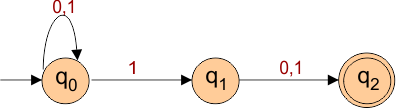### Step 02: Draw NFA transition Table.

NFA transition table of above NFA is given below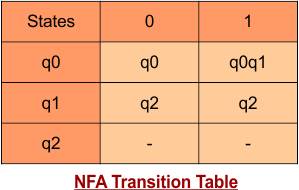### Step 03: Now convert NFA transition Table To its corresponding DFA transition Table

First consider the first row of NFA transition table which will becomes the first row of DFA transition table. Its given below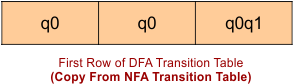We can see in above row, transition for q0 is already found but the q0q1 is new state. If any new state comes into picture then we will find the transition for that state. As

δ'([q0q1], 0) = δ(q0, 0) ∪ δ(q1, 0)

= {q0} ∪ {q2}

= [q0q2]  // new state generated

δ'([q0q1], 1) = δ(q0, 0) ∪ δ(q1, 1)

= {q0q1} ∪ {q2}

= {q0q1q2}  // new state generated

So the second row of DFA transition table will becomes as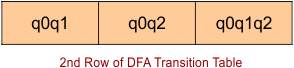We can see in above second row of DFA transition table, the new states q0q2 and q0q1q2 occurs. We need to find the transitions for new generated states.

q0q2 becomes the third row and q0q1q2 will be the 4th row of DFA table. First find the 3rd row.

δ'([q0q2], 0) = δ(q0, 0) ∪ δ(q2, 0)

= {q0}

= [q0]   // already found in DFA table

δ'([q0q2], 1) = δ(q0, 0) ∪ δ(q2, 1)

= {q0q1}

= {q0q1}

= [q0q1]  // already found in DFA table

So the third row is given below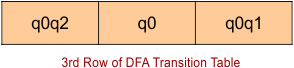Now find the 4th row which is q0q1q2.

δ'([q0q1q2], 0) = δ(q0, 0) ∪ δ(q1, 0)  ∪ δ(q2, 0)

= {q0} ∪ {} ∪ {q2}

= [q0q2]   // already found in DFA table

δ'([q0q1q2], 1) = δ(q0, 1) ∪ δ(q1, 1)  ∪ δ(q2, 1)

= {q0} ∪ {q1} ∪ {q2}

= [q0q1q2]  // already found in DFA table

So, the 4th row is given below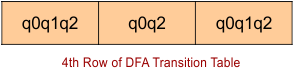At this stage, all generated states are executed for their transitions. So, DFA table is ready.

Now combine all four rows as given below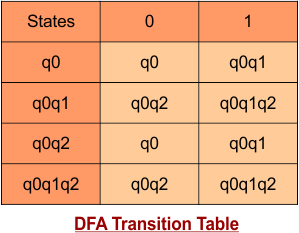### Step 4: Now draw DFA according to above transition table as below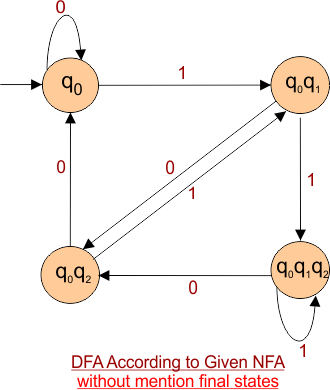### Step 05: Double circle the final state

As q2 was the final state in NFA, So all those states will be the final in DFA where q2 exist. Therefore the DFA with all its final states is given under,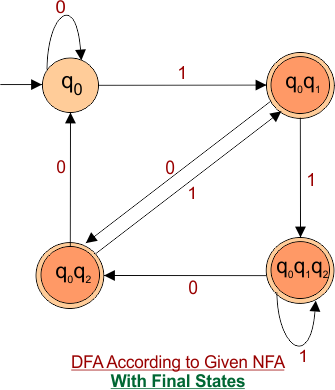Note: Hence proved that,

DFA has higher number of states as compare to NFA states. If “n” is the maximum number of states in NFA then 2n is the maximum number of states to its corresponding DFA.

Help Other’s By Sharing…